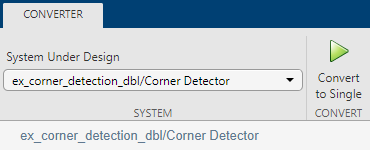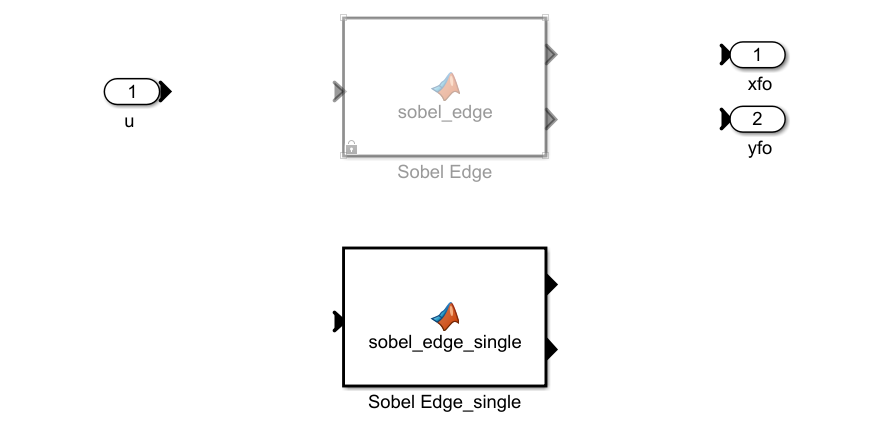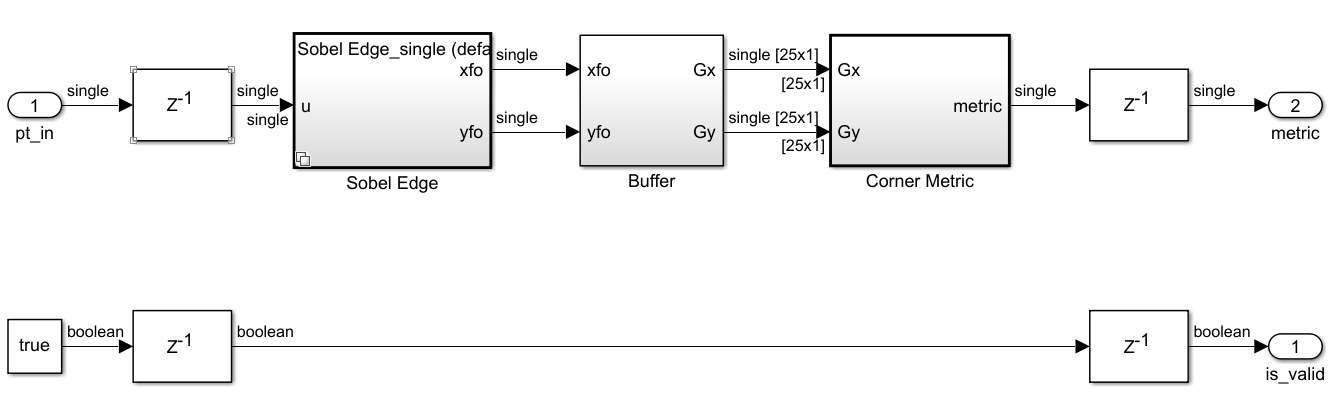## Convert a System to Single Precision

This example shows how to convert a system to single precision using the Single Precision Converter. This example converts a subsystem of a double-precision model to single precision. To convert a subsystem in a model to single precision, surround the subsystem under design with Data Type Conversion blocks before opening the Single Precision Converter.

1. Open the model. At the command line, enter

```addpath(fullfile(docroot,'toolbox','fixpoint','examples')) ex_corner_detection_dbl```The model uses a combination of double-precision, Boolean, and built-in integer data types.

2. Open the Single Precision Converter. From the Simulink® Apps tab, select Single Precision Converter.

3. Under System Under Design, select the system or subsystem to convert to single precision. For this example, select the ```Corner Detector``` subsystem. Click .The converter first checks the system for compatibility with the conversion and changes any model settings that are incompatible. The language standard of the model must be set to C99 (ISO), and the model must use a fixed-step solver.

The converter converts the system and lists all converted data types. The converter changes only double-precision data types. It does not convert Boolean, fixed-point, or built-in integer types to single precision.

When the system under design contains a MATLAB® Function block, the converter creates a variant subsystem containing a generated single-precision version of the MATLAB Function block and the original MATLAB Function block.In the final stage of the conversion, the Converter verifies that the conversion was successful by updating the model.4. Return to the model and update the diagram. The blocks inside the Corner Detector subsystem no longer use double-precision data types.